The MboxWave Wyckoff Trading System for NinjaTrader consists of the following indicators. Each section will explain all of the indicator options.

## MBoxChart

This indicator is what draws the boxes on the chart. They are drawn in real time and the data calculated in the data box also happens
in real time. The boxes are a mini wave, and allow you to see them on the chart really well.

MBoxChart Example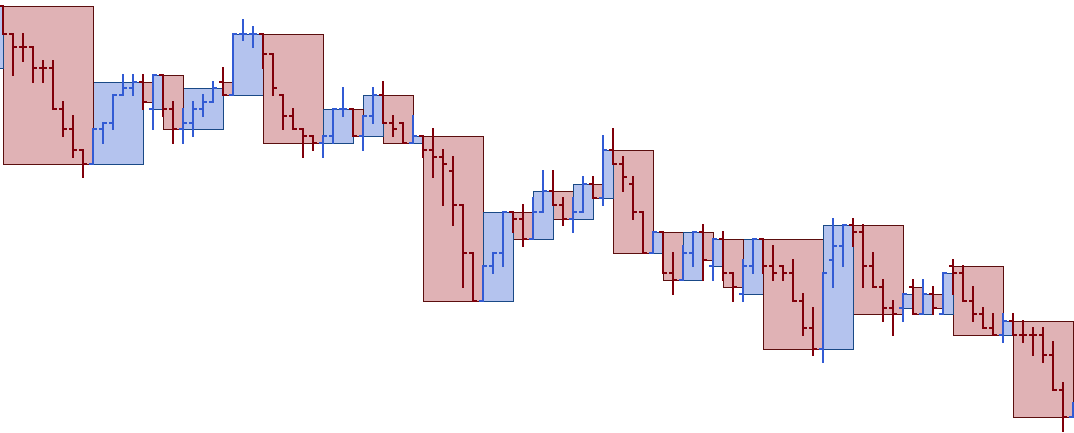A box encompasses upbars or downbars. They are mini waves. The main characteristics of a box are height and width. The height represents the length of the wave. The width has a correlation to either volume or time, depending on the type of chart we are looking at. If you use a volume chart then the width will equal the volume. If using a tick chart the width shows how much effort (number of bars in box) were drawn to form that wave and the progress made. The boxes are a really good way to visually see which way the market is going. When there are more red boxes that are longer and bigger than the blue boxes, price tends to go down. When there are more blue boxes, the opposite is true.

MBoxChart Data Box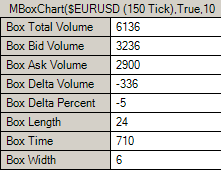When you hover over any box with the mouse cursor, you will see all the information about that box in the data box.

MBoxChart Parameters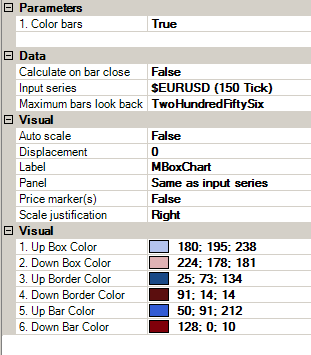1. Color Bars - If set to true this will color the bars in the boxes using Up Bar Color and Down Bar Color 2. Calculate on bar close - The boxes are calculated in real time if set to false. All the info in the Data Box is populated in real time. 3. You can set all the colors for the boxes and bars in the Visual Section.

## MBoxHistogram

This indicator draws the histogram that correlates to the boxes drawn by MboxChart.

MBoxHistogram Example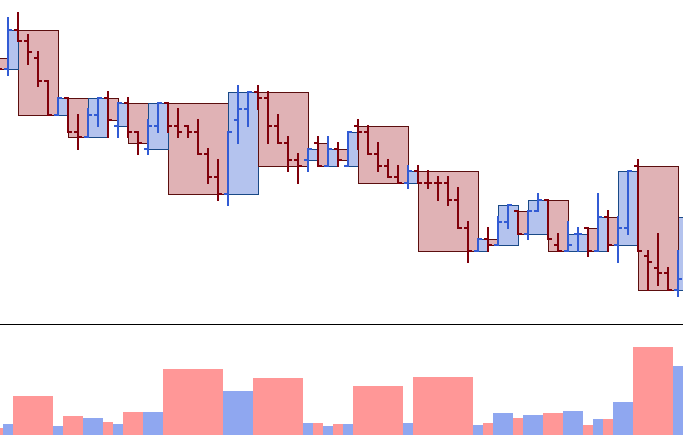MBoxHistogram Data Box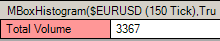This will show you what the histogram is displaying and its value

MBoxHistogram Parameters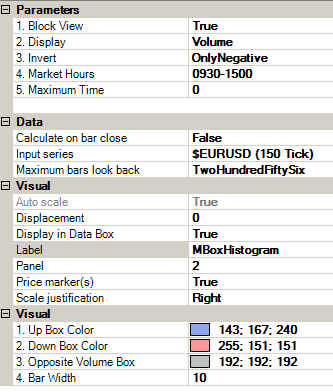1. Block View - If set to true, this will draw the histogram in blocks. If set to false, will draw the histogram in steps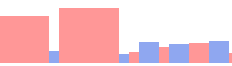2. Display - You can choose what the histogram will show. You can choose from Volume, Bid Volume, Ask Volume, Delta Volume, Delta Percent, Length, Time, Width. 3. Invert        False: Does nothing.        True: Inverts the histogram (Shows histogram upside down).        Only Negative: Will invert negative Delta Volume or negative Delta Percent.               Example viewing Delta Volume, Invert set to False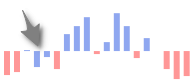Example viewing Delta Volume, Invert set to Only Negative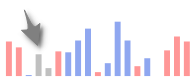It should be noticed , where the grey arrow is pointing that there is negative delta        volume on an upbox. When Invert is set to Only Negative and we have        negative delta volume on an upbox, or positive delta volume on a downwave,        those bars in the histogram will be painted grey to be able discern this. This        color can be changed in "Opposite Volume Box". 4. Market Hours - This only applies when viewing Time. Setting a time range for  market hours will filter out calculating time when outside this range. This is very convenient so that we dont see huge time waves outside normal or busy trading times.        Example. Market Hours set to 0, will not filter out time collection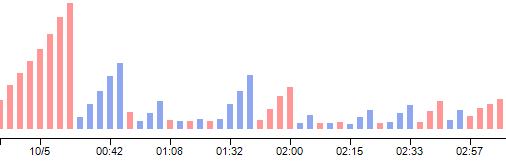Example: Market Hours set to 0100-1500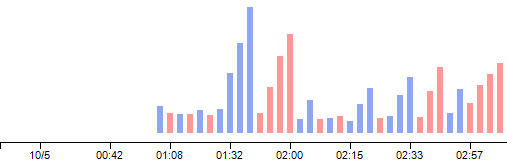5. Maximum Time. Also filters time. If time for a wave exceeds set amount in seconds, time will stop calculating for that wave.

## MWaveChart

This indicator draws the wave lines up and down and can display up to 2 different pieces of data on each wave.

MWaveChart Example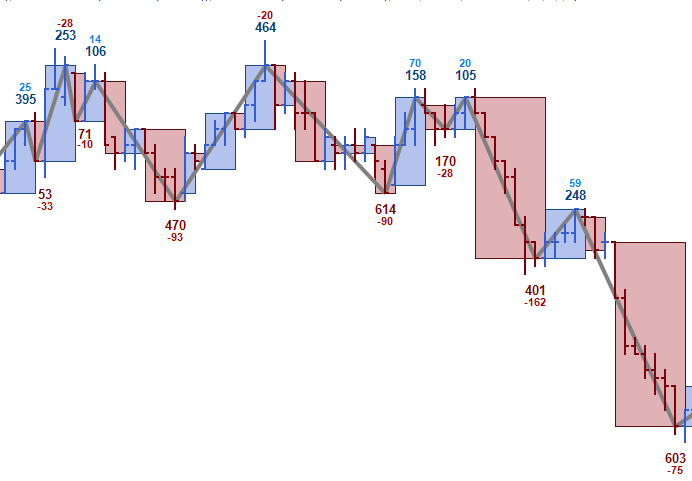In this example the primary label shows volume and the second label shows delta volume. We see that preceding the sharp down turn, there are 2 waves that have more selling than buying going on at the -28 and -20 (delta volume). Supply comes in at 614 volume and shortly after that the market begins to move down.

MWaveChart Data Box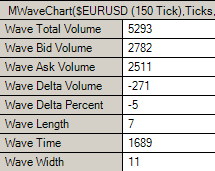When you hover over any wave with the mouse cursor, you will see all the information about that wave in the data box.

MWaveChart Parameters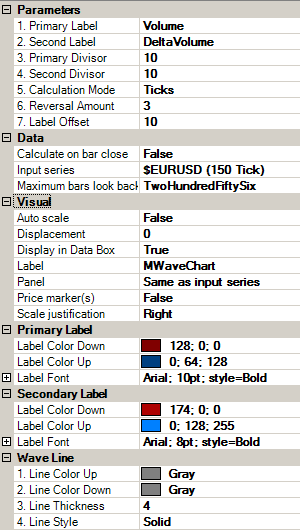1. Primary Label - Can show Volume, Bid Volume, Ask Volume, Delta Volume, Delta Percent, Length, Time, Width. 2. Second Label - Can show Volume, Bid Volume, Ask Volume, Delta Volume, Delta Percent, Length, Time, Width. 3. Primary Divisor - If you want to show the number shown by the label in a more tidy manner, use the divisor. 4. Second Divisor - If you want to show the number shown by the label in a more tidy manner, use the divisor. 5. Calculation Mode - Use Ticks or Percent to calculate wave formation. 6. Reversal Amount - Enter the number in Ticks or Percent to determine when a new wave forms.        Example: For a 3 tick reversal wave enter "3". For a 5% percent reversal wave enter "5". 7. Label Offset. Adjust the offset of the labels drawn in pixels. And you can adjust all the visuals parameters for the labels and waves.

## MWaveHistogram

This indicator draws the histogram that correlates to the zig zags.

MWaveHistogram Example

Showing wave volume in the histogramMWaveHistogram Data BoxThis will show you what the histogram is displaying and its value

MWaveHistogram Parameters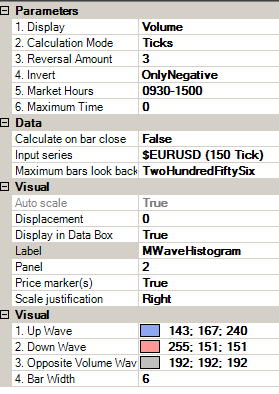1. Display - You can choose what the histogram will show. You can choose from Volume, Bid Volume, Ask Volume, Delta Volume, Delta Percent, Length, Time, Width. 2. Calculation Mode - Use Ticks or Percent to calculate wave formation. 3. Reversal Amount - Enter the number in Ticks or Percent to determine when a new wave forms.        Example: For a 3 tick reversal wave enter "3". For a 5% percent reversal wave enter "5". 4. Invert        False: Does nothing.        True: Inverts the histogram (Shows histogram upside down).        Only Negative: Will invert negative Delta Volume or negative Delta Percent.               Example viewing Delta Volume, Invert set to FalseExample viewing Delta Volume, Invert set to Only NegativeIt should be noticed , where the grey arrow is pointing that there is negative delta        volume on an upwave. When Invert is set to Only Negative and we have        negative delta volume on an upwave, or positive delta volume on a downwave,        those bars in the histogram will be painted grey to be able discern this. This        color can be changed in "Opposite Volume Wave". 5. Market Hours - This only applies when viewing Time. Setting a time range for  market hours will filter out calculating time when outside this range. This is very convenient so that we dont see huge time waves outside normal or busy trading times.        Example. Market Hours set to 0, will not filter out time collectionExample: Market Hours set to 0100-15006. Maximum Time. Also filters time. If time for a wave exceeds set amount in seconds, time will stop calculating for that wave.

MBidAskVolume uses an algorithm to compute Bid/Ask and Delta Volume since NinjaTrader 7 does not provide this data. This is achieved by examining the price movement of every single tick, and then classifying it as Bid/Ask to then calculate the Delta volume.  The result is very close to native Bid/Ask and Delta. This indicator must be loaded for any delta calculations (DeltaVolume, DeltaPercent, MCumulativeDelta) to work.

## MAutoFloorCeiling

This indicator has one main setting. The period controls how many previous bars are analyzed when drawing the automatic support and resistance lines. A smaller period will draw more lines. A larger period will draw less, but they will be longer. Check out the page MAutoFloorCeiling Indicator for how to use this indicator.  Fibonacci numbers tend to be good periods. You can try 8, 13, 21, 34, 55.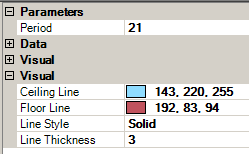## MCumulativeDelta

This indicator has one main setting. The period controls how many previous bars are analyzed when determining the cumulative delta in the histogram. A smaller period will cause the histogram to react faster. A larger period will have more smoothing and a slower reaction in the histogram. Check out the page MCumulativeDelta Indicator for how to use this indicator. Some numbers to try for periods can be 13, 20, 55, 200 (long term).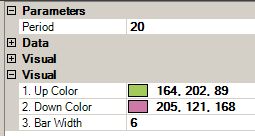## MPace

Check out the page MPace Indicator for how to use this indicator.